# 一文带你了解 Python 中的生成器

• 2022 年 3 月 29 日
• 本文字数：2896 字

阅读完需：约 10 分钟## 前言

def yrange(n):    i = 0    while i < n:        yield i        i += 1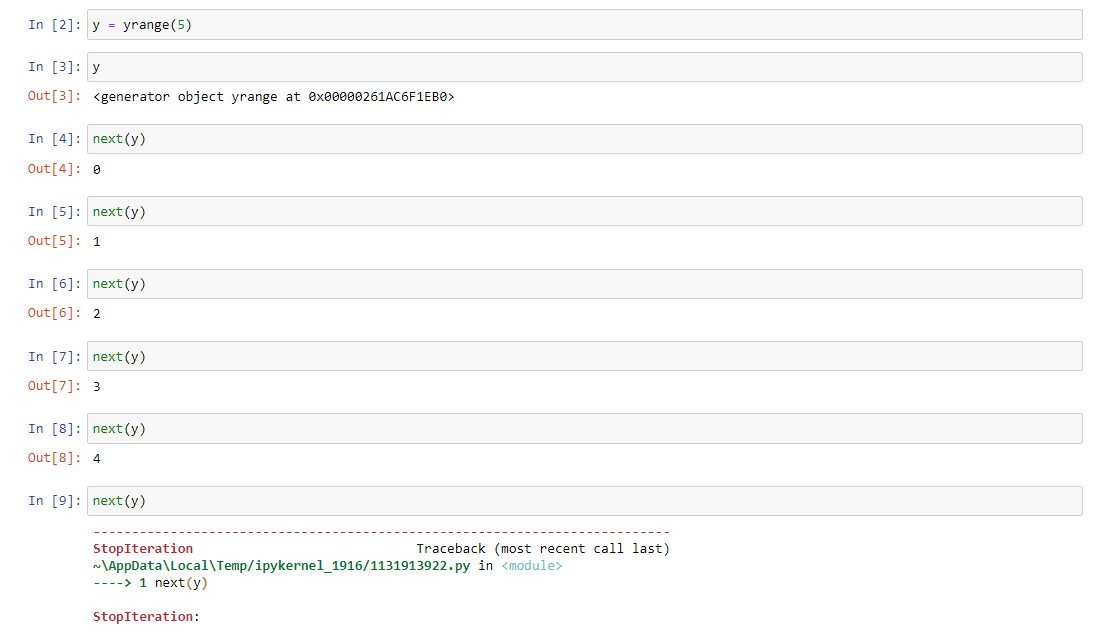“生成器”这个词被混淆地用来表示生成的函数和它生成的内容。

>>> def foo():...     print("begin")...     for i in range(3):...         print("before yield", i)...         yield i...         print("after yield", i)...     print("end")...>>> f = foo()>>> next(f)beginbefore yield 00>>> next(f)after yield 0before yield 11>>> next(f)after yield 1before yield 22>>> next(f)after yield 2endTraceback (most recent call last):  File "<pyshell#13>", line 1, in <module>    next(f)StopIteration>>>

## 生成器也是迭代器

f_2 = foo()for i in f_2: print(i)beginbefore yield 00after yield 0endbefore yield 11after yield 1endbefore yield 22after yield 2end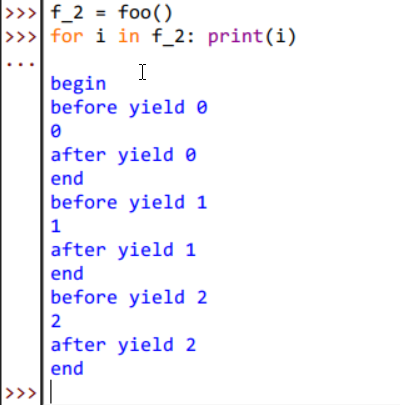def yrange():    i = 1    while True:        yield i        i = i + 1def squares():    for i in yrange():        yield i * idef take(n, seq):    seq = iter(seq)    result = []    try:        for i in range(n):            result.append(next(seq))    except StopIteration:        pass    return resultprint(take(5, squares()))# [1, 4, 9, 16, 25]

def fib(n):    if n <= 1:        return 1    a, b = 0, 1    for _ in range(n):        a, b = b, a + b        yield afor i in fib(10):    print(i, end=' ')# Result：1 1 2 3 5 8 13 21 34 55 

## 生成器推导式

• 使用 yield 会产生一个生成器对象

• 用 return 将返回当前的第一个值。

generator_expressions = (x for x in range(10))generator_expressions<generator object <genexpr> at 0x0000023F8BC51AF0>sum(generator_expressions)45

## 无限生成器

a = range(5)print(list(a))[0, 1, 2, 3, 4]

def infinite_sequence():    num = 0    while True:        yield num        num += 1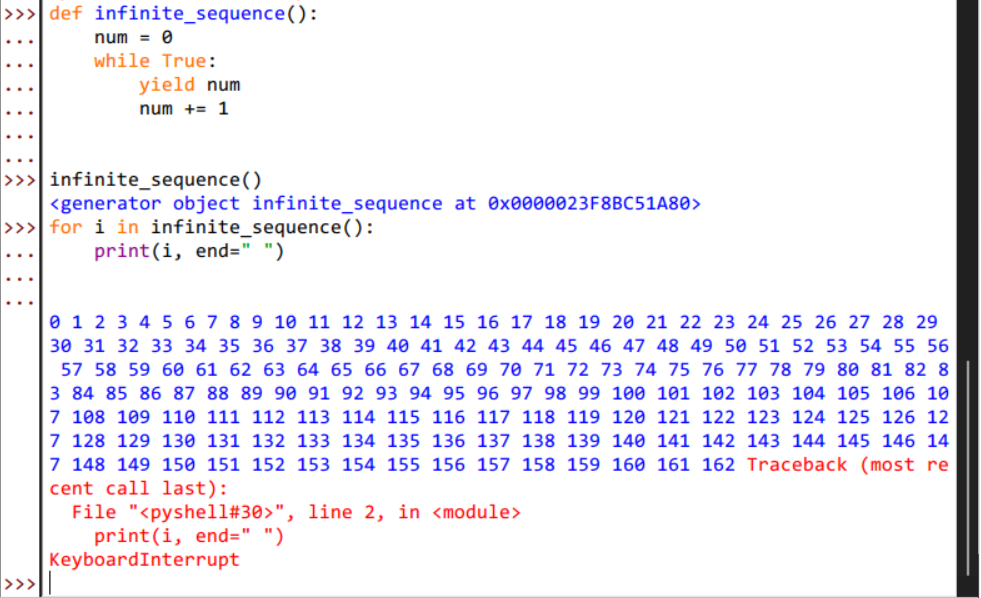## 生成器实际用法

1. 读取文件行

def csv_reader(file_name):    file = open(file_name)    result = file.read().split("\n")    return resultcsv_gen = csv_reader("some_file.csv")row_count = 0for row in csv_gen:    row_count += 1print(f"Row count is {row_count}")

def csv_reader(file_name):    for row in open(file_name, "r"):        yield row

1. 读取文件内容

def readfiles(filenames):    for f in filenames:        for line in open(f):            yield linedef grep(pattern, lines):    return (line for line in lines if pattern in line)def printlines(lines):    for line in lines:        print(line, end="")def main(pattern, filenames):    lines = readfiles(filenames)    lines = grep(pattern, lines)    printlines(lines)

## 高级生成器用法

• send() 函数

• throw() 函数

• close() 函数

1. 首先，新建一个生成器将生成素数，其实现如下：

def isPrime(n):    if n < 2 or n % 1 > 0:        return False    elif n == 2 or n == 3:        return True    for x in range(2, int(n**0.5) + 1):        if n % x == 0:            return False    return Truedef getPrimes():    value = 0    while True:        if isPrime(value):            i = yield value            if i is not None:                value = i        value += 1

1. 然后我们调用 send() 函数，这个函数会向生成器 prime_gen 传入一个值，然后从这个值开始计算下一个素数的值：

prime_gen = getPrimes()print(next(prime_gen))print(prime_gen.send(1000))print(next(prime_gen))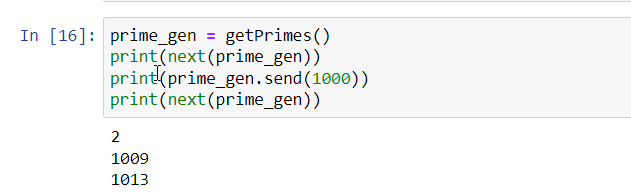1. throw() 允许您使用生成器抛出异常。例如，这对于以某个值结束迭代很有用。比如我们想得到小于 20 的素数就可以使用如下方法：

prime_gen = getPrimes()for x in prime_gen:    if x > 20:        prime_gen.throw(ValueError, "I think it was enough!")    print(x)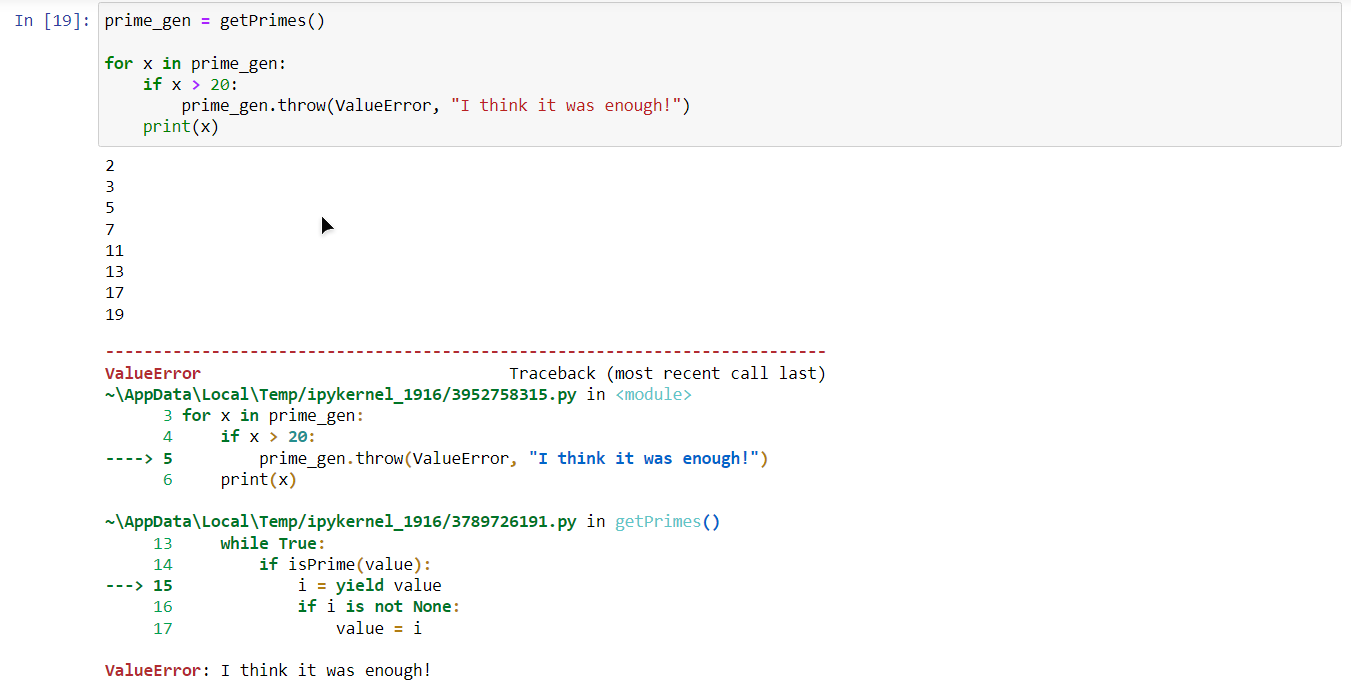1. 在前面的示例中，我们通过引发异常来停止迭代，但这并不是用户想看到的，谁想看到报错呢。因此，结束迭代的更好方法是使用 close():

prime_gen = getPrimes()for x in prime_gen:    if x > 20:        prime_gen.close()    print(x)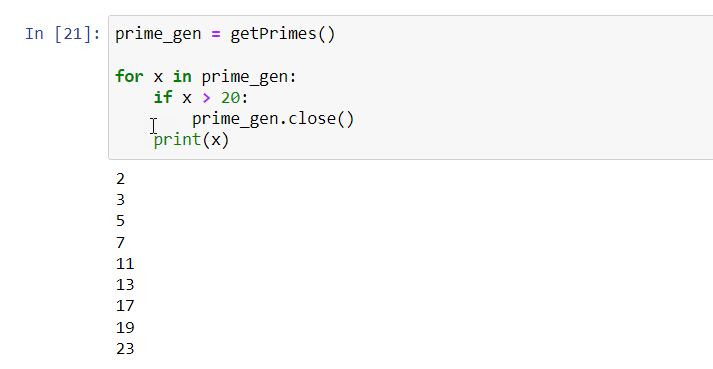## 总结

• https://realpython.com/introduction-to-python-generators/

• https://anandology.com/python-practice-book/iterators.html## 评论